# How to Sort Data by Last Character in Excel

This post will guide you how to sort cells by last character in Excel. How do I sort data by the last character with a formula in Excel. How to sort cells based on the last character with User Defined Function in Excel.

## Sorting Data by Last Character with Formula

Assuming that you have a list of data in range B1:B5, in which contain text string values. And you need to sort cells based on the last characters in cells. We can easily sort cells by the first character, but there is no function or command to sort cells by the last character directly in Microsoft Excel. How to do it. You can use a helper column and then use a formula based on the RIGHT function to extract the last character, then sort helper column, and the column B also be sorted. Here are the steps:

#1 enter the following formula down a nearby column B.

`=RIGHT(B1,1)`

#2 press Enter key on your keyboard, and then drag the Fill Handle down to other cells.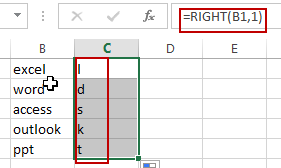#3 you need to keep selecting the cells in helper column, and go to DATA tab, click Sort A to Z command under Sort & Filter group. Then the Sort Warning dialog will open. Select Expand the selection option in the Sort Warning dialog box. Click Sort button.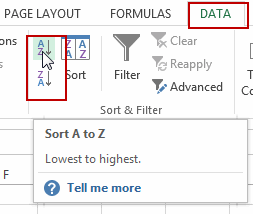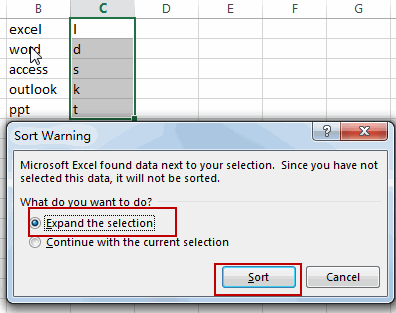#4 you should notice that the range of cells have been sorted. And now you can delete the helper column.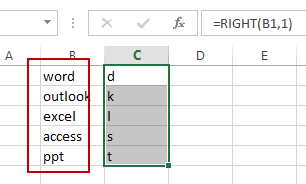## Sorting Data by Last Character with User Defined Function

There is another method to sorting Data by the last character with User Defined Function in Excel. You can try to reverse the text string in Cells, and the last character of original text string will become the first character. So that you can directly sort the cells by the Sort command in the helper column. Here are the steps:

#1 open your excel workbook and then click on “Visual Basic” command under DEVELOPER Tab, or just press “ALT+F11” shortcut.#2 then the “Visual Basic Editor” window will appear.

#3 click “Insert” ->”Module” to create a new module.#4 paste the below VBA code into the code window. Then clicking “Save” button.

```Public Function ReverseString(R As Range)
ReverseString = StrReverse(R.Text)
End Function```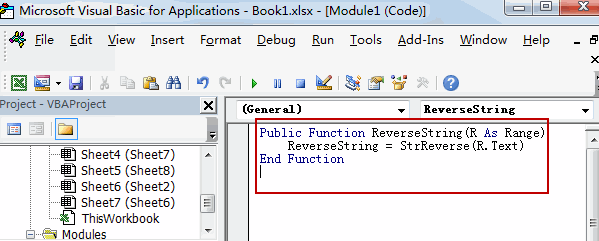#5 back to the current worksheet, then type the following formula in a blank cell, and then press Enter key.

`=ReverseString(B1)`

#6 drag the Fill Handle down to other cells.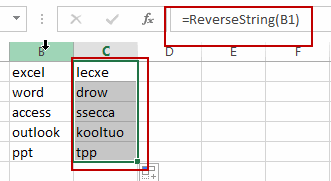#7  keep selecting the cells in helper column, and go to DATA tab, click Sort A to Z command under Sort & Filter group. Then the Sort Warning dialog will open. Select Expand the selection option in the Sort Warning dialog box. Click Sort button.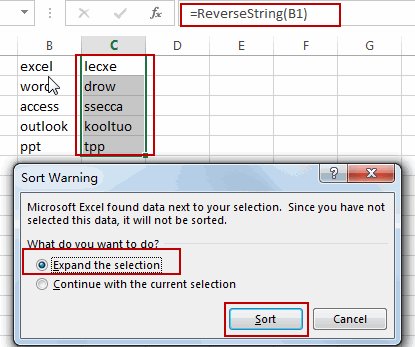#8 you should notice that the range of cells have been sorted. And now you can delete the helper column.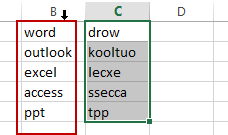### Related Functions

• Excel RIGHT function
The Excel RIGHT function returns a substring (a specified number of the characters) from a text string, starting from the rightmost character.The syntax of the RIGHT function is as below:= RIGHT (text,[num_chars])…

Related Posts

How to Extract First Letter from Each Word in a Cell in Excel

This post will guide you how to extract first letter from each word in a given cell in Excel. How do I extract the first letter of each word in a range of names in your current worksheet in Microsoft ...

How to ignore Blank Cells while Concatenating cells in Excel

This post will guide you how to concatenate cells but ignore all blank cells in your worksheet in Excel. How do I concatenate cells but ignore blank cells with a formula in Excel. How to create a concatenate formula to ...

How to Calculate the Average Excluding the Smallest & Highest Numbers in Excel

Calculating the average for a batch of data is frequently used in our daily life. But for some cases like statistic the average score in a competition, or price analysis, we often calculate the average excluding the smallest and highest ...

How to Get the Maximum or Minimum Absolute Value in Excel

It is easy to find the maximum or minimum value in a batch of data in excel, but if this batch of numbers contains both positive and negative numbers, the maximum or minimum absolute value cannot be found out by ...

How to Count Only Numbers in Bold in a Range of Cells in Excel

This post will guide you how to Count only numbers with bold style in a range of cells in Excel. How do I Count on cells with bold font within a range of cells using User defined function in Excel ...

How to Sum Only Numbers in Bold in a Range of Cells in Excel

This post will guide you how to sum only numbers with bold style in a range of cells in Excel. How do I sum on cells with bold font within a range of cells using User defined function in Excel ...

How to Filter Formula in Excel

This post will guide you how to filter your data with an Formula in Excel. How do I filter cells with formulas through a User defined function in Excel 2013/2016. Filter Formula Assuming that you have a list of data ...

How to Count Cells by Font Color in Excel

This post will guide you how to count cells by font color in Excel. How do I Count the number of cells with specific cell Font color by using a User Defined function in Excel. Count Cells by Font Color ...

How to Highlight Cells Containing Formulas in Excel

This post will guide you how to highlight cells in which contain formulas using Conditional Formatting feature in Excel. How do I conditionally format a cell if it contains formula using a User defined function in combination with Conditional Formatting ...

How to Extract Number from Text String in Excel

This post will guide you how to extract number from a given test string in Excel. How do I extract all numbers from string using a formula in Excel. How to get all number from a given test string using ...

Sidebar